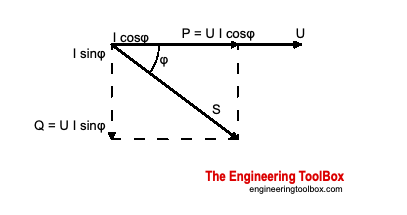Engineering ToolBox - Resources, Tools and Basic Information for Engineering and Design of Technical Applications!

# AC - Active, Reactive and Apparent Power

## Real, imaginary and apparent power in AC circuits.

The required power supply to an electric circuit depends on the

• active power - real electrical resistance power consumption in circuit
• reactive power - imaginary inductive and capacitive power consumption in circuit

The required power supply is called the apparent power and is a complex value that can be expressed in a Pythagorean triangle relationship as indicated in the figure below.### Apparent Power - S

The apparent power is the power supplied to the electric circuit - typical from a power supplier to the grid - to cover the real and reactive power consumption in the loads.

Apparent power can be calculated as

S = (Q2 + P2)1/2                     (1)

where

S = apparent power supply to the circuit (volt ampere, VA)

Q = reactive power consumption in load (volt ampere reactive, VAR)

P = active power consumption in load (watts, W)

Apparent power is measured in volt-amperes (VA) - the AC system voltage multiplied with flowing current. Apparent power is a complex value and the vector sum of the active and reactive power as indicated in the figure above.

#### Single Phase Current

S = U I                  (2a)

where

U = electric potential (V)

I = current (A)

#### Three Phase Current

S = 31/2 U I

= 1.732 U I                 (2b)

### Active Power - P

Active - or Real or True - power do the actual work in the load. Active power is measured in watts (W) and is the power consumed by electrical resistance.

• True power is the current in phase with the voltage multiplied with the voltage

#### Single Phase Current

P = U I cos φ

= U I PF                   (3a)

where

φ = phase angle between the electrical potential (voltage) and the current

PF = cos φ

=  Power Factor

#### Three Phase Current

P = 31/2 U I cos φ

= 1.732 U I PF                     (3b)

#### Direct Current

P = U I                         (3c)

### Reactive Power - Q

Reactive power is the imaginary or complex power in a capacitive or inductive load. Reactive power represents an energy exchange between the power source and the reactive loads where no net power is gained or lost. The net average reactive power is zero. Reactive power is stored in and discharged by inductive motors, transformers, solenoids and capacitors. A pure inductor and a pure capacitor do not consume any power since in a half cycle whatever power is received from the source by these components, the same power is returned to the source.

Reactive power should be minimized because it increases the overall current flowing in an electric circuit without providing any work to the load. Increased reactive currents only provides unrecoverable power loss due to power line resistance.

Increased reactive and apparent power will devrease the power factor - PF.

Reactive inductive power is measured in volt-amperes reactive (VAR).

• Reactive power is the current out of phase with the voltage multiplied with the voltage

#### Single Phase Current

Q = U I sin φ        (4a)

where

φ = phase angle

#### Three Phase Current

Q = 31/2 U I sin φ

=  1.732 UI sin φ                   (4b)

## Related Topics

• ### Electrical

Electrical units, amps and electrical wiring, wire gauge and AWG, electrical formulas and motors.

## Related Documents

• ### AC Circuits - Power vs. Voltage and Current

The alternating current In an AC circuit is generated by a sinusoidal voltage source.
• ### Aluminum Conductor Characteristics

Characteristics of all-aluminum conductors (AAC).
• ### Complex Numbers

Complex numbers are used in alternating current theory and mechanical vector analysis.
• ### Electric Circuit Diagram - Drawing Template

Online shareable electric circuit diagram.
• ### Electric Current vs. Power - Single and Three Phase AC

Convert between single phase (120, 240 and 480 Voltage) and three phase (240 and 480 Voltage).
• ### Electric Motor Calculator

Calculate amps, hp and kVA for electrical motors.
• ### Law of Cosines

One side of a triangle when the opposite angle and two sides are known.
• ### Single Phase Power Equations

Power equations for single phased electrical systems.
• ### Three-Phase Electrtical Motors - Power Factor vs. Inductive Load

Inductive loads and power factors with electrical three-phase motors.
• ### Three-Phase Power Equations

Electrical 3-phase equations.

## Engineering ToolBox - SketchUp Extension - Online 3D modeling!

Add standard and customized parametric components - like flange beams, lumbers, piping, stairs and more - to your Sketchup model with the Engineering ToolBox - SketchUp Extension - enabled for use with older versions of the amazing SketchUp Make and the newer "up to date" SketchUp Pro . Add the Engineering ToolBox extension to your SketchUp Make/Pro from the Extension Warehouse !

We don't collect information from our users. More about

## Citation

• The Engineering ToolBox (2005). AC - Active, Reactive and Apparent Power. [online] Available at: https://www.engineeringtoolbox.com/kva-reactive-d_886.html [Accessed Day Month Year].

Modify the access date according your visit.

9.19.12